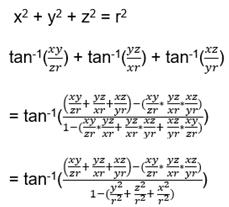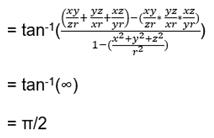# Test: Inverse Trigonometry (Competition Level) -2

## 30 Questions MCQ Test Mathematics (Maths) Class 12 | Test: Inverse Trigonometry (Competition Level) -2

Description
This mock test of Test: Inverse Trigonometry (Competition Level) -2 for JEE helps you for every JEE entrance exam. This contains 30 Multiple Choice Questions for JEE Test: Inverse Trigonometry (Competition Level) -2 (mcq) to study with solutions a complete question bank. The solved questions answers in this Test: Inverse Trigonometry (Competition Level) -2 quiz give you a good mix of easy questions and tough questions. JEE students definitely take this Test: Inverse Trigonometry (Competition Level) -2 exercise for a better result in the exam. You can find other Test: Inverse Trigonometry (Competition Level) -2 extra questions, long questions & short questions for JEE on EduRev as well by searching above.
QUESTION: 1

Solution:
QUESTION: 2

### If cot-1 x + cot-1 y + cot-1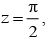then x + y + z is equal to

Solution: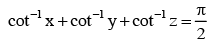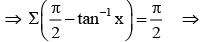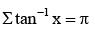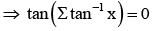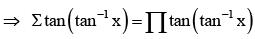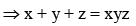QUESTION: 3

###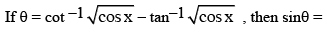Solution:
QUESTION: 4

If a, b, c be positive real numbers and the value of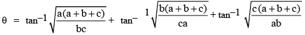then tanθ is equal to -

Solution: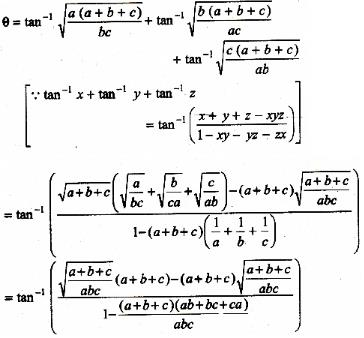=> θ = tan-1 0
=> tan θ = 0

QUESTION: 5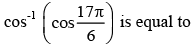Solution: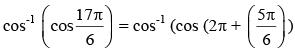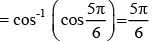QUESTION: 6

The value of  tan–1(1) + cos–1(–1/2) + sin–1(–1/2) is equal to -

Solution:

Since, here we are considering only principle solutions

tan-1(1) = π/4

cos-1(-1/2) = 2π/3

sin-1(-1/2) = -π/6

Sol : π/4 +2π/3 -π/6

: 3π/4

QUESTION: 7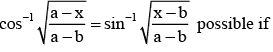Solution:

L.H.S. and R.H.S. of the given equation are defined if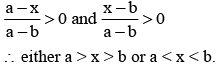QUESTION: 8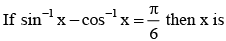Solution: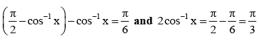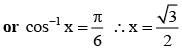QUESTION: 9

Value of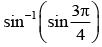Solution: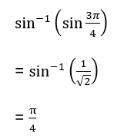QUESTION: 10

The Greatest value among tan1, tan-11, sin1, sin-11, cos1

Solution:

Greatest value sin-11 = π/2

QUESTION: 11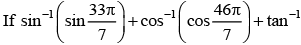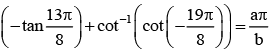Where a and b are in their lowest form, then (a + b) equal to

Solution:

On solving, we get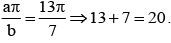QUESTION: 12

If domain of function f:x→x² + 1 is {0,1}, then its range is

Solution:
QUESTION: 13

The sum of the series cot–12 + cot–18 + cot–118 + cot–132 + ….. is

Solution: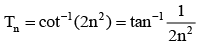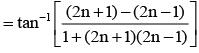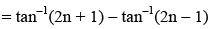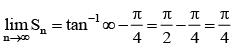QUESTION: 14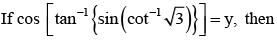Solution:

From the given equation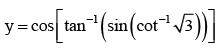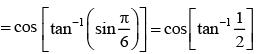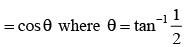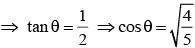[we take only the +ve sign before the square root since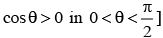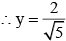QUESTION: 15

If tan (x + y) = 33 and x = tan–1 3, then y will be

Solution:

tan (X + Y) = 33 --- eqn1
X = tan–1 3
So,
tan X = 3
eqn1 ⇒ 33 = (tanX + tanY)/(i-tanX*tanY)
33 = ( 3 + tanY )/( 1 – 3*tanY)
33 – 99*tanY = 3 + tanY
30 = 100 * tanY
tanY = 0.3
So, Y = tan-1(0.3)

QUESTION: 16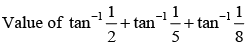Solution: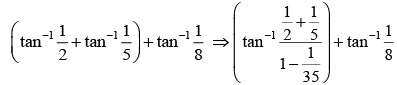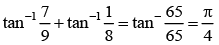QUESTION: 17

The number of solutions of the equation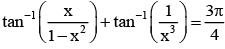belonging to the interval ( 0,1) is

Solution: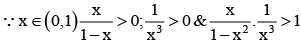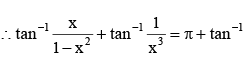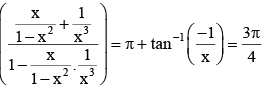⇒ x = 1(not possible)

QUESTION: 18

The value of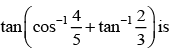Solution: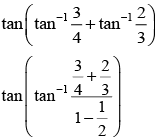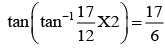QUESTION: 19

If cos-1 x - cos-1 y/2 = α, then 4x2 − 4xy cos α + y2 is equal to

Solution: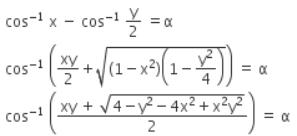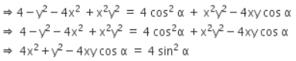QUESTION: 20

If [sin-1 cos-1 sin-1 tan-1x] = 1, ëû where [.] denotes the greatest integer function, then x belongs to the interval.

Solution:

[x] = K, K ∈ Z ⇒ K < x < K + 1, where [x] represents integer part of x.

QUESTION: 21

The value of sin-1 [cos(cos-1(cosx) + sin-1 (sinx))], where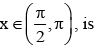Solution:

sin-1 [cos(cos-1(cosx) + sin-1 (sinx))],   where x€(π/2,π)

=>  sin-1[cos(x + π-x)]
=>  sin-1[cos(π)]
=>  sin-1[-1]
=>  -π/2

QUESTION: 22

The principal value of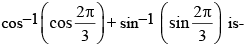Solution: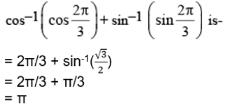QUESTION: 23

If  x1, x2 , x3 , x4 are roots of the equation x4 - x3 sin 2β + x2 cos2β - x cosβ - sinβ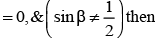Tan-1 (x1) + Tan-1 (x2) + Tan-1 (x3) + Tan-1(x4) can be equal to

Solution: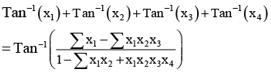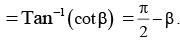QUESTION: 24

The number of solutions of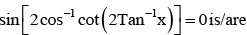Solution:

Explanation : sin(2cos-1(cot(2tan-1x)))=0

2cos-1(cot(2tan-1x))=sin-10=0

cos-1(cot(2tan-1x))=0

cot(2tan-1x)=cos0

cot(2tan-1x)=1

cot(tan-1(2x/(1−x2)))=1

(1−x2)/2x=1

1−x2=2x

x2+2x−1=0

x=[−2±√(4+4)]/2

x=−1±√2, +-1

QUESTION: 25

If xy + yz + zx = 1, then, tan–1x + tan–1y + tan–1z =

Solution:

If xy + yz + zx = 1,
Then, 1 – xy – yz – zx = 0 ----- eqn1
tan-1x + tan-1y = tan-1((x+y)/(1-xy))
tan-1x + tan-1y + tan-1z = tan-1((x+y)/(1-xy)) + tan-1z
= tan-1( ( ((x+y)/(1-xy)) + z ) / (1 – ((x+y)*z)/(1+xy)))
= tan-1((x+y+z-xyz)/(1-xy-yz-zx))
= tan-1((x+y+z-xyz)/0)  [From eqn1]
= π/2

QUESTION: 26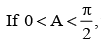then the value of tan-1 (sin A) + tan-1(sin3 A) + tan-1 (cot A cos A)

Solution: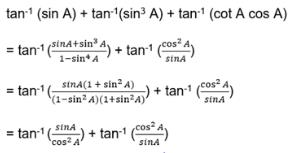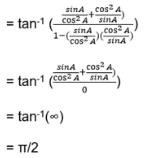QUESTION: 27

The equation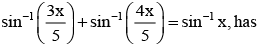Solution: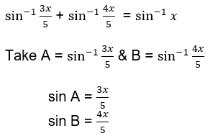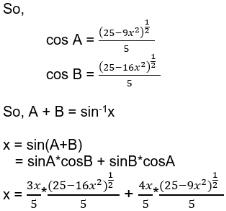Cancelling x both sides (One root x=0)
25 = 3*(25-16x2)1/2 + 4*(25-9x2)1/2
25 - 3*(25-16x2)1/2 = 4*(25-9x2)1/2
Squaring both sides,
625 + 9*(25-16x2) – 2*25*3*(25-16x2)1/2 = 16*(25-9x2)
625 + 225 – 144x2 – 2*25*3*(25-16x2)1/2 = 400 – 144x2
450 = 2*25*3*(25-16x2)1/2
3 = (25-16x2)1/2
Squaring both sides
9 = 25 – 16x2
x2 = 1
x = ±1
So, roots of the equation are
x = -1, 0, 1
Sum of the roots is zero.

QUESTION: 28

The number of real solution of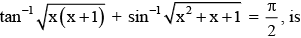Solution:

From function it is clear that
(1) x(x + 1) > 0    ∴ Domain of square root function.
(2) x2 + x + 1>0   ∴ Domain of square root function.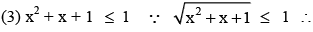Domain of sin-1 function. From (2) and (3)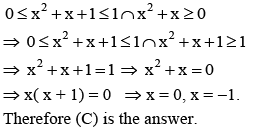QUESTION: 29

If 0 < x < 1, the numberof solutions of the equation tan-1(x-1) + tan-1 x + tan-1 (x+1) = tan-1 3x is

Solution:

The given equation can be written as
tan-1(x-1) + tan-1 (x +1) = tan-1 3x - tan-1 x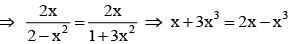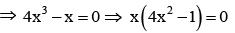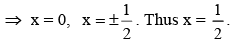QUESTION: 30

If x2 + y2 + z2 = r2, then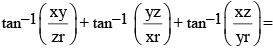Solution: